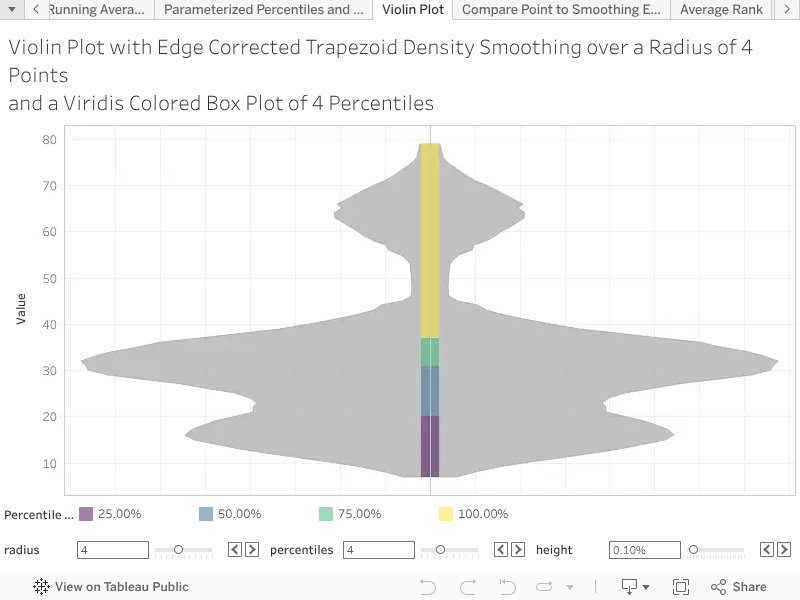# Violin Plots from Record Counts with Dynamic Smoothing and Percentiles

Version 9

Description:

Violin plots are used to compare distributions on the same continuous dimension, particularly when working with sparse data. A violin plot combines a density estimator and a box plot to allow for both a qualitative and quantitative comparison of distributions. In previous articles we have described how to account for edge artefacts when smoothing, ensure consistency with respect to trapezoid integration, create contiguous filled bar charts, and generate dynamic percentiles from record counts. In this article we tie all those techniques together to generate a Tableau version of a violin plot. We will assemble the violin plot in 12 steps, using the techniques developed in the previous articles.

1. Create three parameters, a positive integer for the number of percentiles [PERCENTILES], a non-negative integer for the radius of the edge corrected moving average [RADIUS], and a small float for the half width of the box plot [WIDTH], ensure they are all displayed on the worksheet.
2. Create the table calculation for the left justified widths of the contiguous filled bar chart that will be used as the box plot in the centre of the violin plot, [LEFT].
3. Create the table calculation for the dynamic percentiles that will divide the box plot [PERCENTILE], typically initialised at four percentiles.
4. Create the table calculation for the density estimator of the violin plot using the smoothed trapezoid density estimator from the article on edge artefacts, [VIOLIN].
5. For a vertical violin plot place the continuous dimension along which the distribution is plotted [ORDER] in the rows shelf.
6. Place the [VIOLIN] density estimator on the columns shelf select area as it's mark type.
7. Place the box plot [WIDTH] parameter on the columns shelf, select bar chart as it's mark type.
8. Combine [VIOLIN] and [WIDTH] on a synchronised dual axis, ensuring [WIDTH] is in the front.
9. Place the [PERCENTILE] calculation in the marks card of [WIDTH], using any property that will subdivide the box plot, such as detail, colour, label, or tool tip (colour is preferable).
10. Place the [LEFT] justified width calculation in the size property of the mark card for the parameter [WIDTH]. Select fixed width and left justification from the size property.
11. Fade the colour of [WIDTH] to 50% so that the [VIOLIN] can be seen behind.
12. To create the mirrored violin plot, follow steps 5 through 11 with the columns axis reversed.

Example Calculation:

All the required calculations have been covered in previous articles, but in the interest of clarity we will replicate each of the three calculations here.

// The left justified width sets the last width to zero,

// and looks ahead to the next position.

[LEFT] := IFNULL(LOOKUP(ATTR([ORDER]), 1), ATTR([ORDER])) - ATTR([ORDER])

// Compute the individual percentiles from the choice of the number of percentiles.

// Integer conversion needs to be used because ceiling and floor cannot evaluate on

// table expressions.

[PERCENTILE] := IIF(

([PERCENTILES] * RUNNING_SUM(SUM(Number of Records))) % TOTAL(SUM([Number of Records])) = 0,

RUNNING_SUM(SUM(Number of Records))) / TOTAL(SUM([Number of Records])),

INT(1 + [PERCENTILES] * RUNNING_SUM(SUM(Number of Records)) / TOTAL(SUM([Number of Records]))) / [PERCENTILES]

)

// Weight each point in the window average inversely proportional to

// the total length of the axis in which the point is integrated

[VIOLIN] := 2 * WINDOW_SUM(

SUM([Number of Records]) / (

(

IFNULL(LOOKUP(ATTR([ORDER]), 1 + [RADIUS]), LOOKUP(ATTR([ORDER]), LAST()))

) - (

IFNULL(LOOKUP(ATTR([ORDER]), -(1 + [RADIUS])), LOOKUP(ATTR([ORDER])), FIRST())

)

),

) / TOTAL(SUM([Number of Records]))

Inputs and Setup:

Violin plots require a continuous dimension on which to plot the estimator and for deriving the distance between points, in this example the field is called [ORDER]. Additionally the violin plot requires three parameters to enable the dynamic setting of the width of the box plot, the number of percentiles in the box plot, and the degree of smoothing in the density estimator.

1. A positive integer for the number of percentiles, typically one to ten, in this example the parameter is called [PERCENTILES].
2. A non-negative integer for the radius of the edge corrected moving average, typically zero to ten, in this example the parameter is called [RADIUS].
3. A small float for the half width of the box plot, typically between 0% and 0.1% with steps of 0.005%, in this example called [WIDTH].

Violin plots require the the field used as the continuous dimension and for determining distances is the same field that the table calculations address, in this example the field is called [ORDER].

Violin plots are a non-parametric technique, with the box plot providing a quantitative comparison of distributions, and the density estimator providing a qualitative comparison of the distributions. In particular the box plot illustrates the specific alignment, or lack, of the percentiles, while the density estimator illustrates multi-modal tendencies in the data.

Related Functions:

WINDOW_SUM, SUM, LOOKUP, TOTAL, ATTR, IFNULL, MIN, FIRST, LAST